# GMAT & MBA Admissions BlogPerforming mental math calculations faster and more accurately is a surprisingly important element of success on the GMAT.  And for any given ability level, It’s also important to gain confidence in your mental math abilities before taking the GMAT. Confidence is often half the battle. At the same time, practicing, having strategies in your mind, and understanding where and how mental math is important can all breed confidence.

In this article on GMAT mental math, we provide an overview of which sections of the GMAT exam require calculation, how to improve your GMAT mental math skills, and which drills are going to be most helpful in acquiring mental math mastery.

## GMAT Calculation

When it comes to solving math problems on the GMAT, there are two sections to be aware of: quantitative and integrated reasoning. There is no calculator provided or allowed throughout the quantitative section, as GMAC doesn’t value manual math calculation as a critical skill. So, calculations should produce clean results or be approximated.

An interface calculator is provided for the integrated reasoning section, so you may use it as a resource. However, it should only be utilized for clumsy or convoluted calculations.

Strategically, mental math should be the first ability you develop in order to achieve your GMAT goals. It should be prioritized. Beyond using mental math as much as possible on GMAT problems and GMAT practice tests, you can also work on this skill more directly. For example, in order to strengthen this skill, practice with free math-aids.com drills to improve speed and accuracy.

There are strategies you can use to more effectively use mental math when taking the GMAT as well.

### Mental Math Strategies and Tactics

Here are a few tips to quickly improve your mental math skills by using easy factors or multiples and employing long division:

#### Use Easy Factors or Multiples

• Seek factors of 2, 5, 10, 100, and ½
• It’s biology!
• Our two hands, five fingers, and ten toes are the basis of decimals
• Examples include:
• Doubling and halving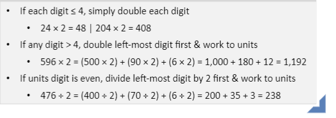• Proportionally manipulating by factors of 10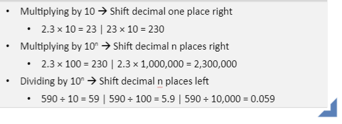• Expedite addition and subtraction by seeking factors of 10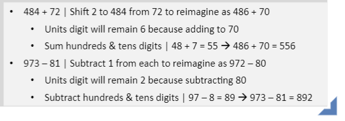• Expedite multiplication by seeking factors of 10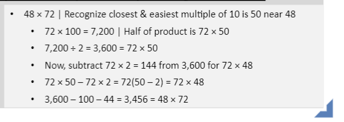• Long Division
• Manipulate manually
• Long division doesn’t actually take that long!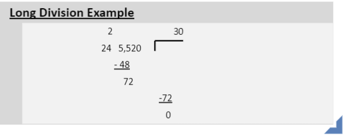### Manual Calculation Drills

We always recommend using math-aids.com as an initial step to elevate your skills. It is a free online resource with dynamically produced worksheets that covers all GMAT calculations topics. A few tips on how to utilize the site for efficient studying:

• Rotate 2-5 Worksheets Daily at Minimum 5-20:00 Total
• Focus on Areas of Difficulty More Frequently
• Practice Both Written Manual Calculation & Mental Math
• Mathematics Warmup Before Any “Real” GMAT Quantitative
• Practice Drills or Exams
• Begin with Top-6 math-aids.com Topics
• Use Search Box to Locate Specific Content Drills Outside Top 6 (ie. Geometry or Word Problems) You Know You Need Work On

### Here are the top 6 math-aids.com topics:

Multiplication

• Times tables advanced timed drills
• Single or multiple digit multiplication worksheets

Division

• Long division worksheets
• Times tables timed drills worksheets

• Exponents with multiplication and division

Fractions

• Adding and subtracting three fractions worksheets
• Multiplying fractions with cross cancelling

Decimals

• Add and subtract worksheets with decimals
• Multiplying by powers of ten with decimals

Percent

• Percentage calculations worksheets
• Word problems - percentage word problems

GMAT mental math tip – memorize times tables and exponents up to 15^2 and 10^3

### Conclusion

In order to reach your GMAT goals, it will be necessary to solve calculations without a calculator, especially throughout the quantitative and integrated reasoning sections.  You will need to integrate mental math practice into your studying, employing tools such as factors, multiples, and long division. A good place to start with mental math drills is math-aids.com, a free resource that covers all GMAT topics. Good luck!